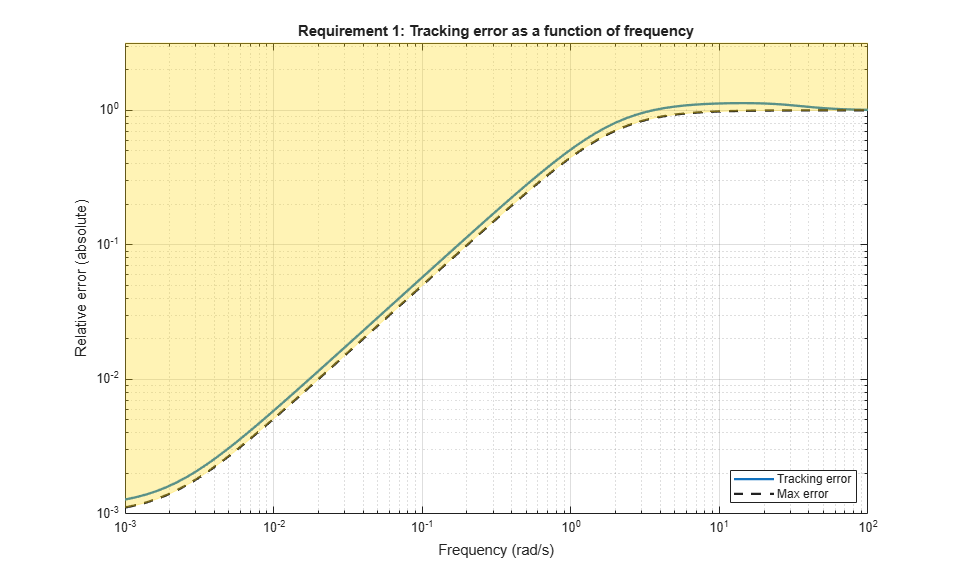# Validating Results

This example shows how to interpret and validate tuning results from `systune`.

### Background

You can tune the parameters of your control system with `systune` or `looptune`. The design specifications are captured using `TuningGoal` requirement objects. This example shows how to interpret the results from `systune`, graphically verify the design requirements, and perform additional open- and closed-loop analysis.

### Controller Tuning with SYSTUNE

We use an autopilot tuning application as illustration, see the "Tuning of a Two-Loop Autopilot" example for details. The tuned compensator is the "MIMO Controller" block highlighted in orange in the model below.

```open_system('rct_airframe2') ```The setup and tuning steps are repeated below for completeness.

```ST0 = slTuner('rct_airframe2','MIMO Controller'); % Compensator parameterization C0 = tunableSS('C',2,1,2); C0.D.Value(1) = 0; C0.D.Free(1) = false; setBlockParam(ST0,'MIMO Controller',C0) % Requirements Req1 = TuningGoal.Tracking('az ref','az',1); % tracking Req2 = TuningGoal.Gain('delta fin','delta fin',tf(25,[1 0])); % roll-off Req3 = TuningGoal.Margins('delta fin',7,45); % margins MaxGain = frd([2 200 200],[0.02 2 200]); Req4 = TuningGoal.Gain('delta fin','az',MaxGain); % disturbance rejection % Tuning Opt = systuneOptions('RandomStart',3); rng('default') [ST1,fSoft] = systune(ST0,[Req1,Req2,Req3,Req4],Opt); ```
```Final: Soft = 1.51, Hard = -Inf, Iterations = 52 Final: Soft = 1.15, Hard = -Inf, Iterations = 87 Final: Soft = 1.15, Hard = -Inf, Iterations = 70 Final: Soft = 1.16, Hard = -Inf, Iterations = 120 ```

### Interpreting Results

`systune` run three optimizations from three different starting points and returned the best overall result. The first output `ST` is an `slTuner` interface representing the tuned control system. The second output `fSoft` contains the final values of the four requirements for the best design.

```fSoft ```
```fSoft = 1.1476 1.1476 0.5461 1.1476 ```

Requirements are normalized so a requirement is satisfied if and only if its value is less than 1. Inspection of `fSoft` reveals that Requirements 1,2,4 are active and slightly violated while Requirement 3 (stability margins) is satisfied.

### Verifying Requirements

Use `viewGoal` to graphically inspect each requirement. This is useful to understand whether small violations are acceptable or what causes large violations. First verify the tracking requirement.

```viewGoal(Req1,ST1) ```We observe a slight violation across frequency, suggesting that setpoint tracking will perform close to expectations. Similarly, verify the disturbance rejection requirement.

```viewGoal(Req4,ST1) legend('location','NorthWest') ```Most of the violation is at low frequency with a small bump near 35 rad/s, suggesting possible damped oscillations at this frequency. Finally, verify the stability margin requirement.

```viewGoal(Req3,ST1) ```This requirement is satisfied at all frequencies, with the smallest margins achieved near the crossover frequency as expected.

### Evaluating Requirements

You can also use `evalGoal` to evaluate each requirement, that is, compute its contribution to the soft and hard constraints. For example

```[H1,f1] = evalGoal(Req1,ST1); ```

returns the value `f1` of the requirement and the underlying frequency-weighted transfer function `H1` used to computed it. You can verify that `f1` matches the first entry of `fSoft` and coincides with the peak gain of `H1`.

```[f1 fSoft(1) getPeakGain(H1,1e-6)] ```
```ans = 1.1476 1.1476 1.1476 ```

### Analyzing System Responses

In addition to verifying requirements, you can perform basic open- and closed-loop analysis using `getIOTransfer` and `getLoopTransfer`. For example, verify tracking performance in the time domain by plotting the response `az` to a step command `azref` for the tuned system `ST1`.

```T = ST1.getIOTransfer('az ref','az'); step(T) ```Also plot the open-loop response measured at the plant input `delta fin`. You can use this plot to assess the classical gain and phase margins at the plant input.

```L = ST1.getLoopTransfer('delta fin',-1); % negative-feedback loop transfer margin(L) grid ```### Soft vs Hard Requirements

So far we have treated all four requirements equally in the objective function. Alternatively, you can use a mix of soft and hard constraints to differentiate between must-have and nice-to-have requirements. For example, you could treat Requirements 3,4 as hard constraints and optimize the first two requirements subject to these constraints. For best results, do this only after obtaining a reasonable design with all requirements treated equally.

```[ST2,fSoft,gHard] = systune(ST1,[Req1 Req2],[Req3 Req4]); ```
```Final: Soft = 1.29, Hard = 0.99959, Iterations = 140 ```
```fSoft ```
```fSoft = 1.2898 1.2898 ```
```gHard ```
```gHard = 0.4674 0.9996 ```

Here `fSoft` contains the final values of the first two requirements (soft constraints) and `gHard` contains the final values of the last two requirements (hard constraints). The hard constraints are satisfied since all entries of `gHard` are less than 1. As expected, the best value of the first two requirements went up as the optimizer strived to strictly enforce the fourth requirement.

```bdclose('all') ```